Courses

# Hydrograph Civil Engineering (CE) Notes | EduRev

## Civil Engineering (CE) : Hydrograph Civil Engineering (CE) Notes | EduRev

The document Hydrograph Civil Engineering (CE) Notes | EduRev is a part of the Civil Engineering (CE) Course Civil Engineering SSC JE (Technical).
All you need of Civil Engineering (CE) at this link: Civil Engineering (CE)

Chapter 6
HYDROGRAPH
Hydrographs: Hydrograph is a response of a given catchment to a rainfall input.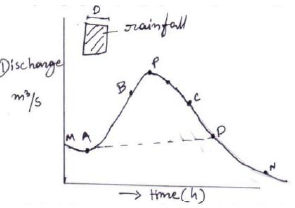Components:
MA= base flow recession, AB= Rising limb, BC= crest segment, CD= falling limb, DN= base flow recession

UNIT HYDROGRAPH:
Unit hydrograph is defined as the hydrograph of direct runoff resulting from 1 unit depth (1cm) of rainfall excess occurring uniformly over the basin and at a uniform rate for a specified duration (D-hours). This called D-hour unit hydrograph.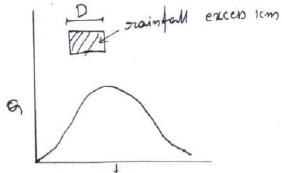Two basic assumptions of unit hydrograph theory:
1. Time  invariance: The  direct  run-off  response  to  a  given  effective  rainfall  in  a catchment is time variant. This implies that the DRH for a given ER in a catchment is always the same irrespective of when it occurs.

2. Linear response: The direct run-off response to the rainfall excess is assumed to be linear. It means that if an input x1 (t)  and causes an output y1(t )and an input x2(t ) causes an output y2 (t), then  input x1(t) + x2(t) gives an output y1(t) + y2 (t).

Application of unit hydrograph: By using unit hydrograph one can easily calculate the DRH. Let it to be assumed that a D-h unit hydrograph and the storm hyetograph are available.
The initial and infiltration losses are estimated and deducted from the storm hyetograph to obtain the ERH. The ERH is then divided into M blocks of D-h duration each. The rainfall excess in each D-h duration is then operated upon the unit hydrograph successively to get the various DRH curves. The ordinates of these DRH’s are suitably lagged to obtain the proper time sequence and are then collected and added at each time to obtain the required net DRH due to the storm

Deviation of unit hydrographs:

1. Method of superposition: If a D h unit hydrograph is available and it is desired to develop a unit hydrograph of nD h where n is an integer, it is easily accomplished by superposing n unit hydrographs with each graph separated from pervious on by D h. The combination of these curves gives n cm rainfall excess. By dividing with n we get nD h unit hydrograph.

2. The S-curve technique: If it is desired to develop a unit hydrograph of duration mD,where m is a fraction, the method of superposition cannot be used. This can be achieved by S-curve. The S-curve, also known as S-hydrograph is a hydrograph produced by a continuous effective rainfall at constant rate for an infinite period. It is a curve obtained by summation of an infinite series of D-h unit hydrographs spaced D hour apart. At any given time the ordinates of the various curves occurring at that time  co-ordinates are summed up to obtain ordinates of S-curve. This S-curve is due to a D-h unit hydrograph. It has a initial steep portion and reaches a maximum equilibrium discharge at a time equal to the time base of the first unit hydrograph. The average intensity of ER producing the S-curve is 1/D cm /h and the equilibrium discharge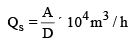Where A= Area of catchment in km2 D= Duration in hours of ER at the unit hydrograph

Derivation of T-hour unit hydrograph: Consider two D-h S-curves A and B displaced T-h. If the ordinates of B are subtracted from that A, the resulting curve is a DRH produced by a rainfall excess of duration T- h and magnitude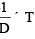cm.
Hence,  if the ordinate  differences  of A and  B i.e. (SA -SB ) are divided by DT , the resulting ordinates denote a hydrograph due to an ER of 1cm and of duration T-h, i.e. a T-h unit hydrograph.

PROBABILITY:
If the probability of an event occurring is P, The probability of the event not occurring is q = 1 - P.
The probability of occurrence of the event r times in n successive years is
pr,n = ncr pr. qn-r
The probability of the event not occurring at all in n successive years is
Po,n = nco po. qn-0 = qn

The probability of the event occurring at least once in a successive years P1 = 1-qn.

The probable maximum precipitation (PMP): It is defined as the greatest or extreme rainfall for a given duration that is physically possible over a station or basin.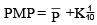Where,K = Precipitation factor.

Evapotranspiration: Evaporation  from  water  bodies  and  soil masses  together  with transpiration from vegetation is known as Evapotranspiration.

Evaporimeters: To estimate the evaporation, evaporimeters are used.

Types of Evaporimeters:
1. Class A evaporation pan
2. ISI standard pan Lake evaporation = Cp ×pan evaporation where Cp = pan coefficient

Transpiration: Transpiration is the process by which water leaves the body of a living plant and reaches atmosphere as water vapour.
Evapotranspiration is measured by Lysimeter.

Evapotranspiration equation:
Penman’s equation: PET=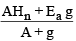Where,
PET= daily potential Evapotranspiration mm/ day
A= slope of saturation vapour pressure temperature curve
Hn= net radiation in mm of evaporable water/ day
Ea = parameters of wind velocity and saturation deficit.
g = constant

Isopleth: The lines on a map through places having equal depths of transpiration are called depth.
Infiltration: Infiltration is the flow of water into the ground through the soil surface. Infiltration capacity:

Horton’s equation:
He observed that the infiltration capacity reduced in an exponential fashion from an initial, maximum rate fcf to a final constant rate fco. Horton expressed the decay of infiltration capacity with time as an exponential decay given by
f = fc + (fo - fc ) e-kt

Where,
f = infiltration capacity at any time t from the start of the rainfall
fo = initial infiltration capacity at t = 0
fc = final steady state value
td = Duration of rainfall
kh = constant depending on soil.

φ -Index : The  φ- index is the average rainfall above which the rainfall volume is equal to the runoff vol.The φ index is derived from the rainfall hyetograph with the edge of the resulting run- off volume.
φ -Index=(I-R)/24
Where,
R = Runoff in cm from a 24- h rainfall of intensity I cm/day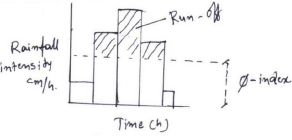W - index :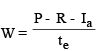P = total storm precipitation
R = total run-off
Ia = initial losses
te = duration of rainfall excess.

Stream flow measurement:
1. Area-velocity method:   Q=A.V
2. Dilution technique
3. Ultrasonic method
4. Indirect method
5. Hydraulic structures
6. Slope area method

Offer running on EduRev: Apply code STAYHOME200 to get INR 200 off on our premium plan EduRev Infinity!

## Civil Engineering SSC JE (Technical)

109 docs|50 tests

,

,

,

,

,

,

,

,

,

,

,

,

,

,

,

,

,

,

,

,

,

;